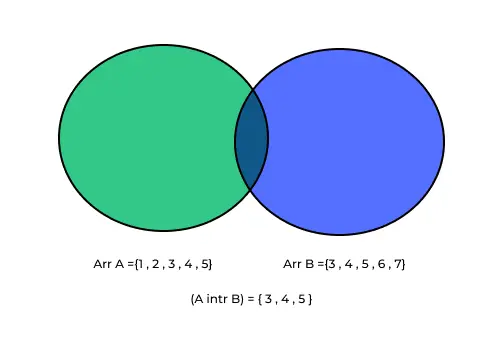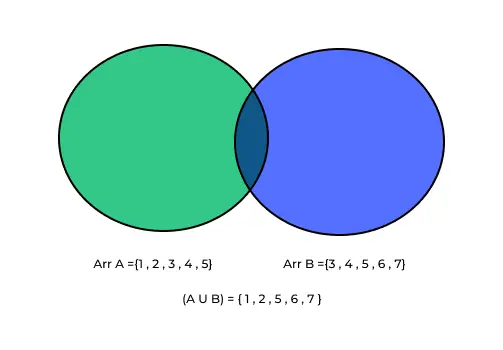# Intersection and Union of Two Array## Intersection and Union of Two sorted Array :

The intersection of two sorted arrays means finding the elements that are common to both arrays. We’ll introduce you to the Naïve, Sorting, and Hashing methods, offering clear insights into when and how to use them effectively. By the end, you’ll be well-equipped to make informed choices based on your data and specific needs.

## What is intersection and union in Python?

1. Intersection in Python:

• Finding common elements in two or more sets or lists.
• Result is a new set (for sets) or list (for lists) with elements present in all input collections.

2. Union in Python:

• Combining elements from multiple sets or lists into a single collection.
• Result is a new set (for sets) or list (for lists) with all unique elements from the input collections.

### How do you implement union and intersection in Python?

Finding the Union of Two Sets:

1.Create two sets you want to find the union of.

`set1 = {1, 2, 3, 4, 5}`

set2 = {3, 4, 5, 6, 7}

2. Use the union() method to find the union of the sets and store the result in a new set.

`union_set = set1.union(set2)`

or

Use the | operator for sets.

`union_set = set1 | set2`

3. The union_set now contains all the unique elements from both set1 and set2.

Finding the Intersection of Two Sets:

1. Create two sets you want to find the intersection of.

`set1 = {1, 2, 3, 4, 5}set2 = {3, 4, 5, 6, 7}`

2. Use the intersection() method to find the intersection of the sets and store the result in   a new set.

`intersection_set = set1.intersection(set2)`

or

Use the & operator for sets.

`intersection_set = set1 & set2`

3. The intersection_set now contains the elements that are common to both set1 and   set2.

### What is intersection?

In the context of sets or arrays, the “intersection” refers to the set of elements that are common to two or more sets or arrays. It represents the elements that exist in both sets. In other words, if you have two sets or arrays, the intersection is the collection of elements that appear in both of them.

For example, if you have two sets:

Set A = {1, 2, 3, 4, 5}

Set B = {3, 4, 5, 6, 7}### What is Union?

In the context of sets or arrays, the “union” refers to the combination of two or more sets or arrays into a single set or array that contains all the unique elements from all the sets. It represents the elements that exist in at least one of the sets.

For example, if you have two sets:

Set A = {1, 2, 3, 4, 5}

Set B = {3, 4, 5, 6, 7}### Approaches For Solving the problem:

Solving the problem of finding the intersection and union of two arrays can be approached in various ways, and here we have discussed them in detail:

### 1.Naïve Approach:

The Naïve approach involves using nested loops to compute the intersection and union. Here’s a Python code example:

```def intersection_union_naive(arr1, arr2):
intersection = []
union = arr1.copy()

for element in arr2:
if element not in union:
union.append(element)
if element in arr1 and element not in intersection:
intersection.append(element)

return intersection, union

arr1 = [1, 2, 3, 4, 5]
arr2 = [3, 4, 5, 6, 7]
intersection, union = intersection_union_naive(arr1, arr2)
print("Intersection:", intersection)
print("Union:", union)
```

### 2.Sorting Approach:

The Sorting approach involves sorting both arrays and then using two pointers to compute the intersection and union efficiently. Here’s a Python code example:

```def intersection_union_sorting(arr1, arr2):
arr1.sort()
arr2.sort()

intersection = []
union = []
i = j = 0

while i < len(arr1) and j < len(arr2):
if arr1[i] == arr2[j]:
intersection.append(arr1[i])
union.append(arr1[i])
i += 1
j += 1
elif arr1[i] < arr2[j]:
union.append(arr1[i])
i += 1
else:
union.append(arr2[j])
j += 1

while i < len(arr1):
union.append(arr1[i])
i += 1

while j < len(arr2):
union.append(arr2[j])
j += 1

return intersection, union

arr1 = [1, 2, 3, 4, 5]
arr2 = [3, 4, 5, 6, 7]
intersection, union = intersection_union_sorting(arr1, arr2)
print("Intersection:", intersection)
print("Union:", union)```

### 3.Hashing Approach:

The Hashing approach uses sets to compute the intersection and union efficiently. Here’s a Python code example:

```def intersection_union_hashing(arr1, arr2):
set1 = set(arr1)
set2 = set(arr2)

intersection = list(set1.intersection(set2))
union = list(set1.union(set2))

return intersection, union

arr1 = [1, 2, 3, 4, 5]
arr2 = [3, 4, 5, 6, 7]
intersection, union = intersection_union_hashing(arr1, arr2)
print("Intersection:", intersection)
print("Union:", union)
```

### Related Banners

Get PrepInsta Prime & get Access to all 200+ courses offered by PrepInsta in One Subscription

## Get over 200+ course One Subscription

Courses like AI/ML, Cloud Computing, Ethical Hacking, C, C++, Java, Python, DSA (All Languages), Competitive Coding (All Languages), TCS, Infosys, Wipro, Amazon, DBMS, SQL and others